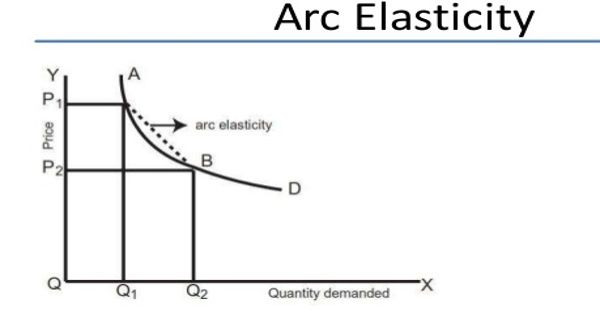Economics

# Arc ElasticityArc elasticity is the elasticity of one variable with respect to another between two given points. In mathematics and economics, the arc elasticity is the elasticity of one variable with respect to another between two given points. It is used when there is no general function to define the relationship between the two variables.

In the concept of arc elasticity, elasticity is measured over the arc of the demand curve on a graph. It measures elasticity between two points on a curve – using a mid-point between the two curves.

Arc elasticity is also defined as the elasticity between two points on a curve. It is the ratio of the percentage change of one of the variables between the two points to the percentage change of the other variable. In economics, there are two possible ways of calculating elasticity of demand—price (or point) elasticity of demand and arc elasticity of demand. It contrasts with the point elasticity, which is the limit of the arc elasticity as the distance between the two points approaches zero and hence is defined at a single point rather than for a pair of points. The arc price elasticity of demand measures the responsiveness of quantity demanded to a price. It takes the elasticity of demand at a particular point on the demand curve, or between two points on the curve. Therefore, the arc elasticity is more useful than the price elasticity when there is a considerable change in price.